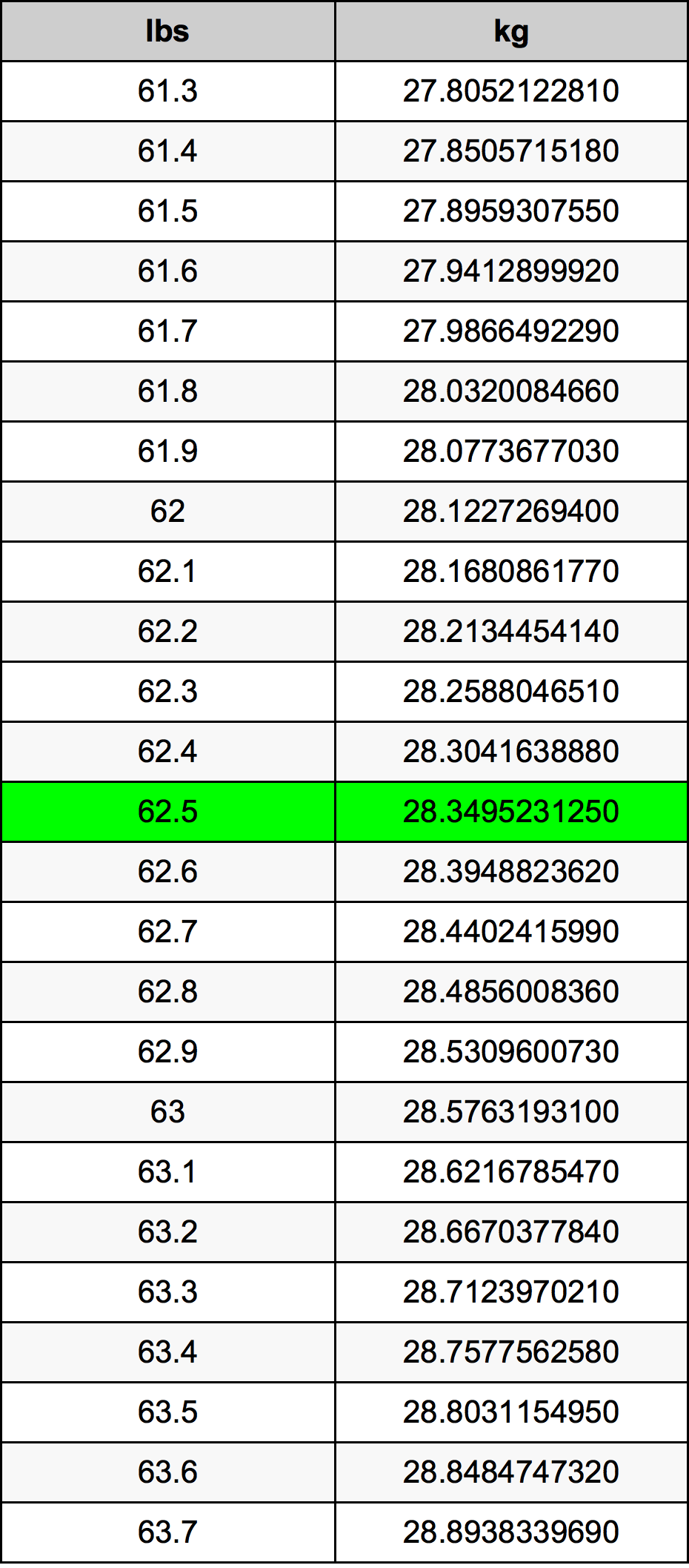Pounds To Kg

# 62.5 lbs to kg62.5 Pounds to Kilograms

lbs
=
kg

## How to convert 62.5 pounds to kilograms?

 62.5 lbs * 0.45359237 kg = 28.349523125 kg 1 lbs
A common question is How many pound in 62.5 kilogram? And the answer is 137.788913866 lbs in 62.5 kg. Likewise the question how many kilogram in 62.5 pound has the answer of 28.349523125 kg in 62.5 lbs.

## How much are 62.5 pounds in kilograms?

62.5 pounds equal 28.349523125 kilograms (62.5lbs = 28.349523125kg). Converting 62.5 lb to kg is easy. Simply use our calculator above, or apply the formula to change the length 62.5 lbs to kg.

## Convert 62.5 lbs to common mass

UnitMass
Microgram28349523125.0 µg
Milligram28349523.125 mg
Gram28349.523125 g
Ounce1000.0 oz
Pound62.5 lbs
Kilogram28.349523125 kg
Stone4.4642857143 st
US ton0.03125 ton
Tonne0.0283495231 t
Imperial ton0.0279017857 Long tons

## What is 62.5 pounds in kg?

To convert 62.5 lbs to kg multiply the mass in pounds by 0.45359237. The 62.5 lbs in kg formula is [kg] = 62.5 * 0.45359237. Thus, for 62.5 pounds in kilogram we get 28.349523125 kg.

## 62.5 Pound Conversion Table## Alternative spelling

62.5 lb to Kilograms, 62.5 lb in Kilograms, 62.5 lb to Kilogram, 62.5 lb in Kilogram, 62.5 lbs to Kilogram, 62.5 lbs in Kilogram, 62.5 Pound to Kilogram, 62.5 Pound in Kilogram, 62.5 lbs to kg, 62.5 lbs in kg, 62.5 lb to kg, 62.5 lb in kg, 62.5 Pounds to Kilograms, 62.5 Pounds in Kilograms, 62.5 Pound to Kilograms, 62.5 Pound in Kilograms, 62.5 Pounds to Kilogram, 62.5 Pounds in Kilogram